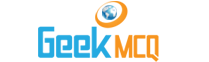Entry Test MCQ :: Waves

@ : Home > Physics > Waves

31.  The particles in the wave separated through __________ multiple of ? are out of phase to each other
 A. (n) B. (n+1) C. (n+1/2) D. both (a) and (c)

32.  Waves produced at the surface of water by a pencil executing vibrating motion if held vertically at a frequency of 50Hz are
 A. Longitudinal B. Transverse C. Periodic D. both (a) and (c)

33.  Newton estimated the speed of sound
 A. 281m/sec B. 333m/sec C. 340m/sec D. all of the above

34.  Laplace found that the alternate compressions and rarefactions produced in sound waves follows
 A. isothermal law B. adiabatic law C. isochoric law D. all of the above

35.  For destructive interference of sound waves the path difference between two interfering sounds should be
 A. n? B. (n+1/2)? C. some time n? and some times (n+1/2)? D. none of these

36.  The speed of sound in hydrogen is __________ time than that in oxygen
 A. Two times B. Three times C. Four time D. Six time

37.  The beats frequency (sensible) for a human car is
 A. 42Hz B. 7 Hz C. 256Hz D. 262Hz

38.  The speed of stationary waves in a stretched string are independent of
 A. Number of loops B. Tension in the string C. Point where string is plucked D. both (a) and (c)

39.  Fundamental frequency of stationary waves in open pipe is ________ times the frequency in closed pipe.
 A. One B. Two C. Four time D. None of these

40.  Which phenomena can be applied to estimate the velocity of star with respect to earth
 A. Dopplers effect B. Interference of waves C. Beats phenomena D. All of these